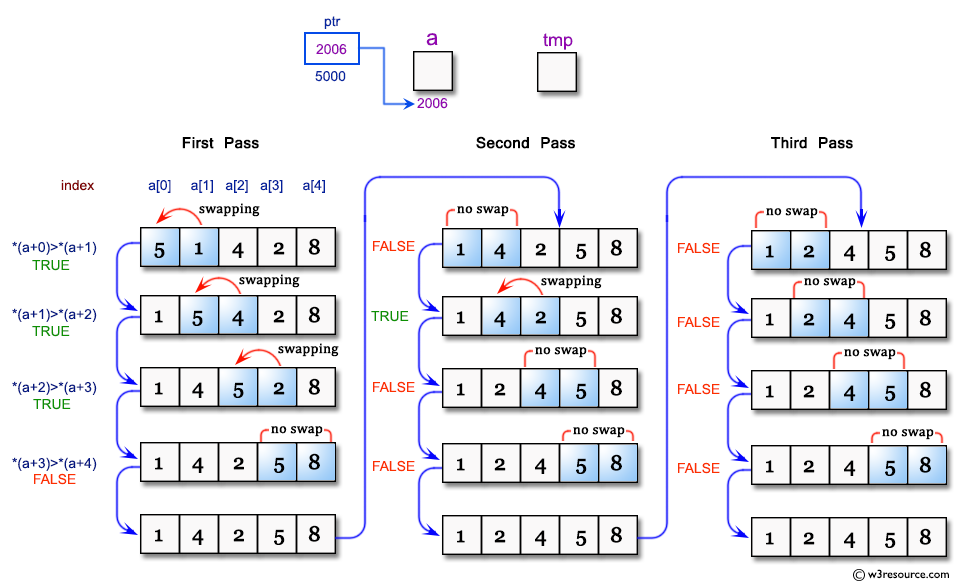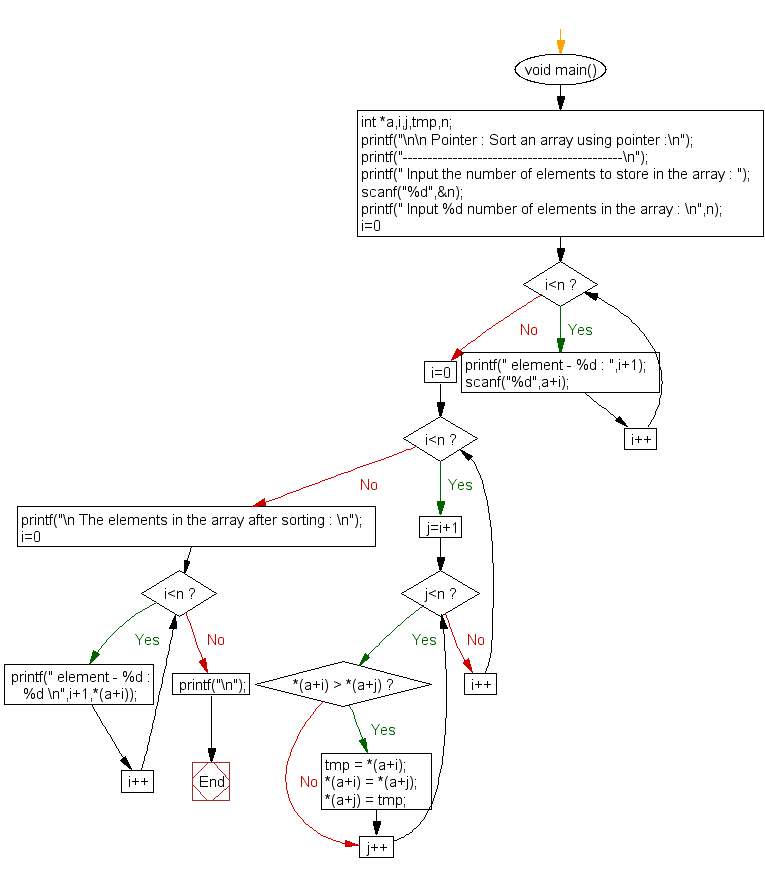﻿ C Program: Sort an array using pointer - w3resource# C Exercises: Sort an array using pointer

## C Pointer : Exercise-14 with Solution

Write a program in C to sort an array using Pointer.

Pictorial Presentation:Sample Solution:

C Code:

``````#include <stdio.h>
void main()
{
int *a,i,j,tmp,n;
printf("\n\n Pointer : Sort an array using pointer :\n");
printf("--------------------------------------------\n");

printf(" Input the number of elements to store in the array : ");
scanf("%d",&n);

printf(" Input %d number of elements in the array : \n",n);
for(i=0;i<n;i++)
{
printf(" element - %d : ",i+1);
scanf("%d",a+i);
}
for(i=0;i<n;i++)
{
for(j=i+1;j<n;j++)
{
if( *(a+i) > *(a+j))
{
tmp = *(a+i);
*(a+i) = *(a+j);
*(a+j) = tmp;
}
}
}
printf("\n The elements in the array after sorting : \n");
for(i=0;i<n;i++)
{
printf(" element - %d : %d \n",i+1,*(a+i));
}
printf("\n");
}
```
```

Sample Output:

```Pointer : Sort an array using pointer :
--------------------------------------------
Input the number of elements to store in the array : 5
Input 5 number of elements in the array :
element - 1 : 25
element - 2 : 45
element - 3 : 89
element - 4 : 15
element - 5 : 82

The elements in the array after sorting :
element - 1 : 15
element - 2 : 25
element - 3 : 45
element - 4 : 82
element - 5 : 89
```

Flowchart :C Programming Code Editor:

Have another way to solve this solution? Contribute your code (and comments) through Disqus.

What is the difficulty level of this exercise?

Test your Programming skills with w3resource's quiz.

﻿

## C Programming: Tips of the Day

How to format strings using printf() to get equal length in the output?

You can specify a width on string fields, e.g.

`printf("%-20s", "initialization..."); `

And then whatever's printed with that field will be blank-padded to the width you indicate.

The - left-justifies your text in that field.

Ref : https://bit.ly/34DMOc3